# Long-Term GDP by EU Region, Second Quarter 2021

Dec 6, 2021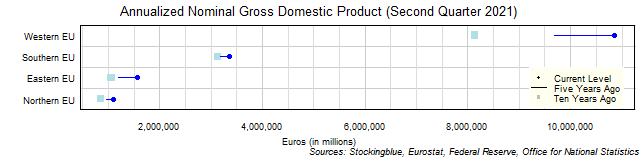The chart above shows the annualized nominal gross domestic product (GDP) in each EU region as of the second quarter of 2021 in millions of euros, the change from five years ago, and the GDP ten years prior.  Every single region's economy grew both over the past five and ten years.

# Long-Term GDP by US Region, Second Quarter 2021

Dec 3, 2021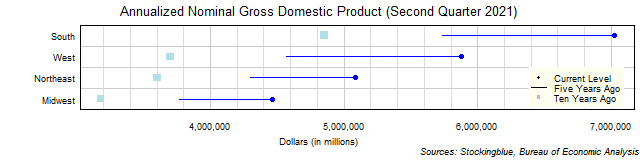The chart above shows the annualized nominal gross domestic product (GDP) in each US region as of the second quarter of 2021 in millions of dollars, the change from five years ago, and the GDP ten years prior.  Every single region's economy grew both over the past five and ten years.

# Long-Term GDP by EU and US State, Second Quarter 2021

Dec 2, 2021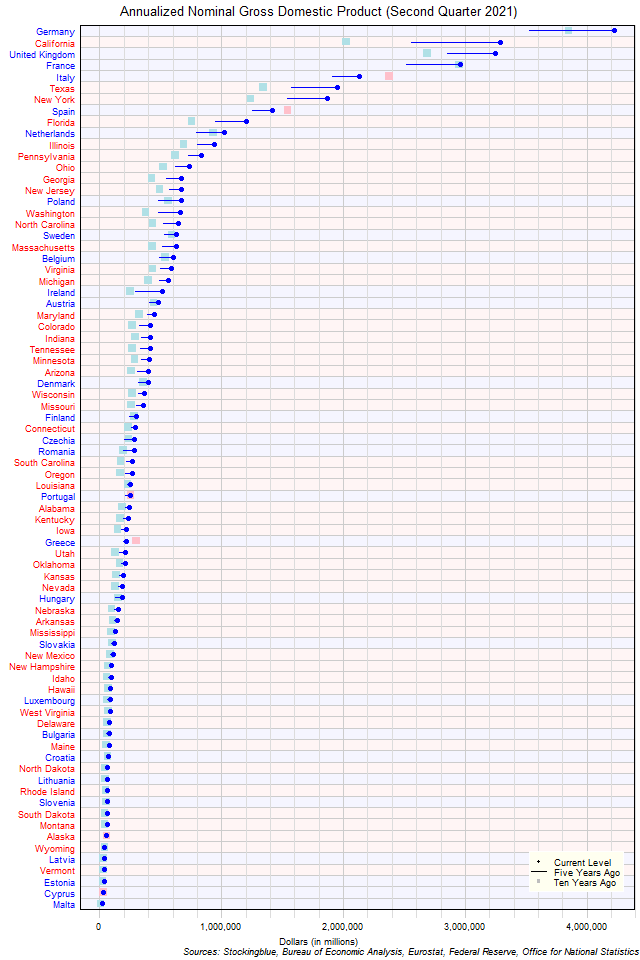The chart above shows the annualized nominal gross domestic product (GDP) in each EU and US state as of the second quarter of 2021 in millions of US dollars, the change from five years ago, and the GDP ten years prior.  Germany continues to be the largest economy.

# Long-Term GDP by EU State, Second Quarter 2021

Dec 1, 2021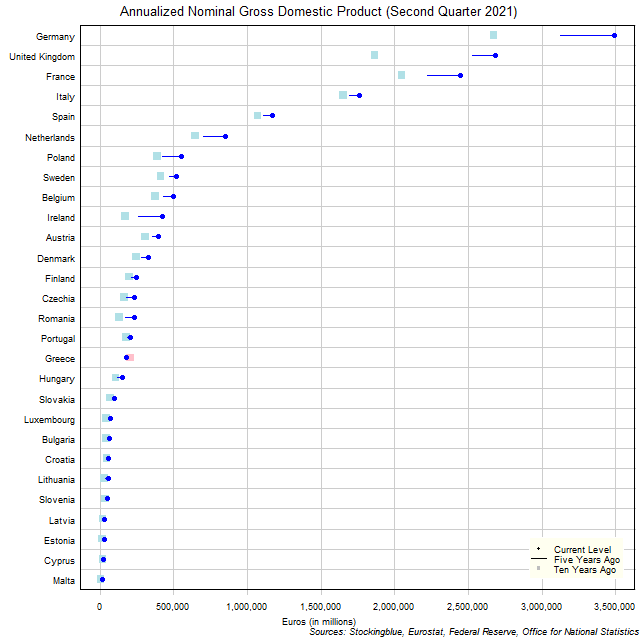The chart above shows the annualized nominal gross domestic product (GDP) in each EU state as of the second quarter of 2021 in millions of euros, the change from five years ago, and the GDP ten years prior.  Germany's economy grew more over the past five years than 17 states' entire GDP.

# Long-Term GDP by US State, Second Quarter 2021

Nov 30, 2021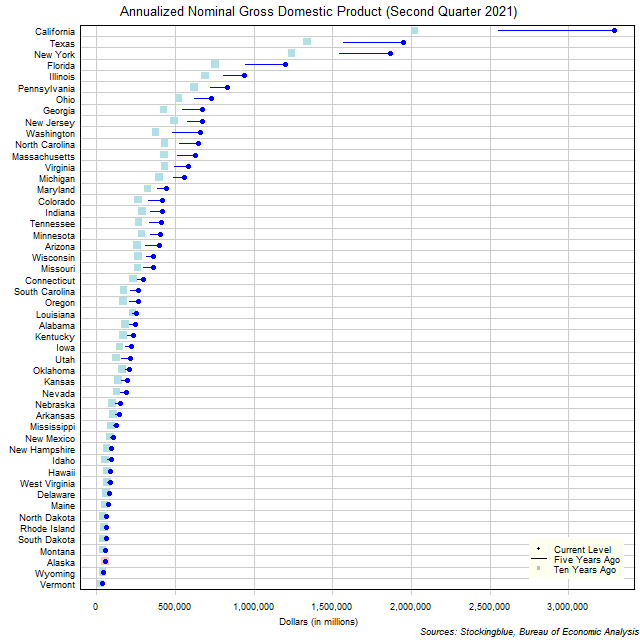The chart above shows the annualized nominal gross domestic product (GDP) in each US state as of the second quarter of 2021 in millions of dollars, the change from five years ago, and the GDP ten years prior.  California's economy grew more over the past five years than the size of 44 states' entire economies.

# EU and US GDP Growth Rate by Region, Second Quarter 2021

Nov 29, 2021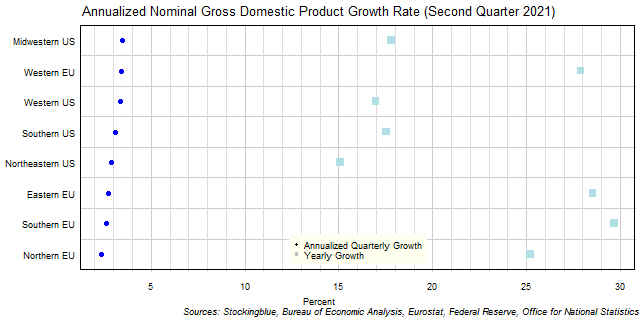The chart above shows the annualized nominal gross domestic product (GDP) quarterly growth rate in each EU and US region as of the second quarter of 2021 and the growth rate from one year prior.  Every single region's economy grew over the previous quarter.

# EU GDP Growth Rate by Region, Second Quarter 2021

Nov 26, 2021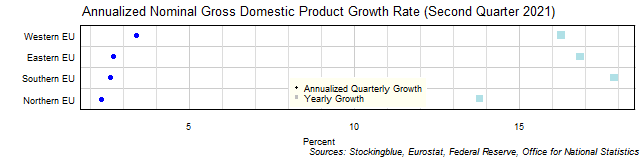The chart above shows the annualized nominal gross domestic product (GDP) quarterly growth rate in each EU region as of the second quarter of 2021 and the growth rate from one year prior.  All four regions grew over the previous quarter.

# US GDP Growth Rate by Region, Second Quarter 2021

Nov 25, 2021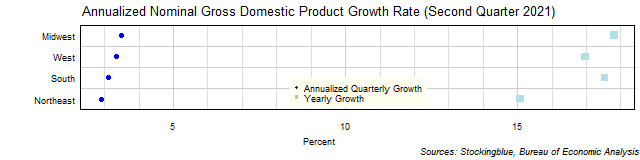The chart above shows the annualized nominal gross domestic product (GDP) quarterly growth rate in each US region as of the second quarter of 2021 and the growth rate from one year prior.  Every single region's economy grew over the previous quarter.

# EU and US GDP Growth Rate by State, Second Quarter 2021

Nov 24, 2021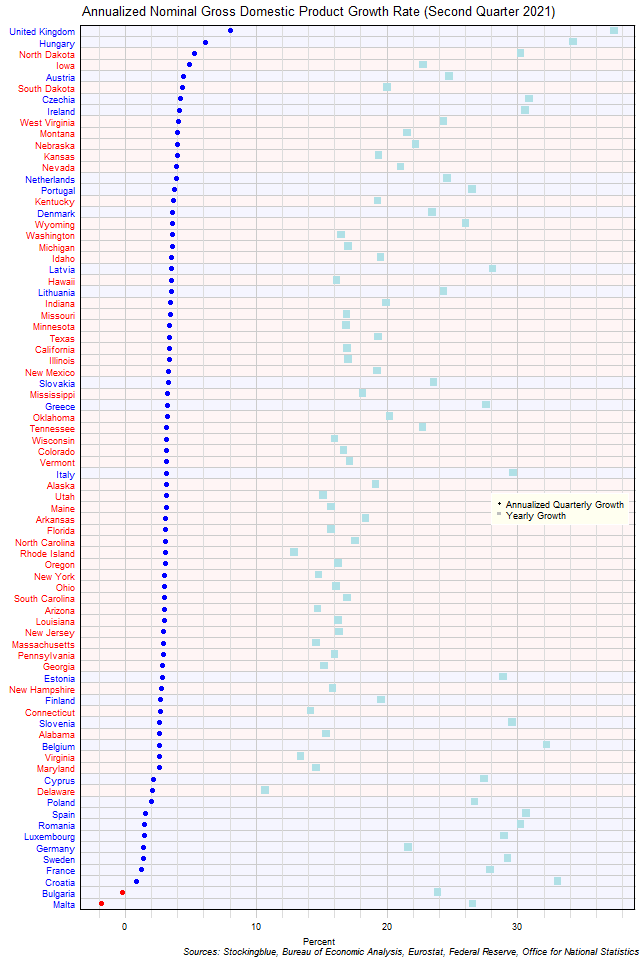The chart above shows the annualized nominal gross domestic product (GDP) quarterly growth rate when the GDP is priced in US dollars in each EU and US state as of the second quarter of 2021 and the growth rate from one year prior.  Bulgaria and Malta were the only states to contract over the previous quarter.

# EU GDP Growth Rate by State, Second Quarter 2021

Nov 23, 2021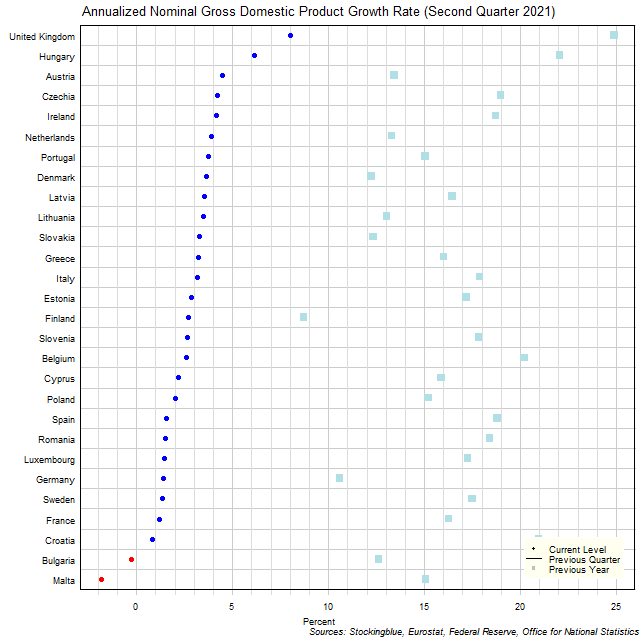The chart above shows the annualized nominal gross domestic product (GDP) quarterly growth rate in each EU state as of the second quarter of 2021 and the growth rate from one year prior.  Two states' economies contracted over the previous quarter.